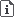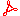FLOC 2022: FEDERATED LOGIC CONFERENCE 2022
PC ON MONDAY, AUGUST 1ST
Days:
previous day
all days

View: session overviewtalk overviewside by side with other conferences

08:30-09:00Coffee & Refreshments
09:00-10:30 Session 26E: Joint QBF Session (joint with QBF)
Location: Ullmann 309
09:00
QBF Solvers and their Proof Systems

ABSTRACT. We give an overview of the main paradigms in QBF solving, as well as techniques for preprocessing. In each case, we present corresponding proof systems and discuss lower bound results. We point to open problems, and consider current trends in solver development.

10:00
PRESENTER: Benjamin Böhm

ABSTRACT. Quantified conflict-driven clause learning (QCDCL) is one of the main approaches for solving quantified Boolean formulas (QBF). We formalise and investigate several versions of QCDCL that include cube learning and/or pure-literal elimination, and formally compare the resulting solving models via proof complexity techniques. Our results show that almost all of the QCDCL models are exponentially incomparable with respect to proof size (and hence solver running time), pointing towards different orthogonal ways how to practically implement QCDCL.

10:30-11:00Coffee Break
11:00-12:30 Session 31H: Joint QBF Session
Location: Ullmann 309
11:00
Strategy Extraction and Proof
12:00
QCDCL with Cube Learning or Pure Literal Elimination – What is best? (II)
12:30-14:00Lunch Break

Lunches will be held in Taub hall and in The Grand Water Research Institute.

14:00-15:30 Session 34I
Location: Ullmann 309
14:00
Hard Inputs from Kolmogorov Randomness---Can Merlin Tame Open Problems?ABSTRACT. We consolidate two widely believed conjectures about tautologies---no optimal proof system exists, and most require superpolynomial size proofs in any system---into a $p$-isomorphism-invariant condition satisfied by all paddable $\textbf{coNP}$-complete languages or none. The condition is: for any Turing machine (TM) $M$ accepting the language, $\textbf{P}$-uniform input families requiring superpolynomial time by $M$ exist (equivalent to the first conjecture) and appear with positive upper density in an enumeration of input families (implies the second). In that case, no such language is easy on average (in $\textbf{AvgP}$) for a distribution applying non-negligible weight to the hard families.

The hardness of proving tautologies and theorems is likely related. Motivated by the fact that arithmetic sentences encoding string $x$ is Kolmogorov random'' are true but unprovable with positive density in a finitely axiomatized theory $\mathcal{T}$ (Calude and J{\"u}rgensen), we conjecture that any propositional proof system requires superpolynomial size proofs for a dense set of $\textbf{P}$-uniform families of tautologies encoding there is no $\mathcal{T}$ proof of size $\leq t$ showing that string $x$ is Kolmogorov random''. This implies the above condition.

The conjecture suggests that there is no optimal proof system because undecidable theories help prove tautologies and do so more efficiently as axioms are added, and that constructing hard tautologies seems difficult because it is impossible to construct Kolmogorov random strings. Similar conjectures that computational blind spots are manifestations of noncomputability would resolve other open problems.

14:30

ABSTRACT. The problem of the existence of an p-optimal propositional proof system is one of the central open problems in proof complexity.

The goal of this work is to study the restricted case of this problem, namely, the case of strong reductions. We also introduce the notion of bounded proof system and study the connection between optimality and automatizability for bounded proof systems.

15:00
PRESENTER: Tomáš Peitl

ABSTRACT. Hitting formulas are a peculiar class of propositional CNF formulas. Not only is their satisfiability decidable in polynomial time, even their models can be counted in closed form. In contrast, other tractable classes, like 2-SAT and Horn-SAT, usually have algorithms based on resolution; and model counting remains hard. On the flip side, those resolution-based algorithms usually easily imply an upper bound on resolution complexity, which is missing for hitting formulas. Are hitting formulas hard for resolution? In this paper we take the first steps towards answering this question. We show that the resolution complexity of hitting formulas is dominated by so-called irreducible hitting formulas. However, constructing large irreducible formulas is non-trivial and it is not even known whether infinitely many exist. Building upon our theoretical results, we implement an efficient algorithm on top of the Nauty software package to enumerate all irreducible unsatisfiable hitting formulas with up to 14 clauses, and we determine exact resolution complexity of those with up to 13 clauses, by extending an existing SAT encoding.

15:30-16:00Coffee Break
16:00-17:30 Session 37I
Location: Ullmann 309
16:00
On vanishing sums of roots of unity in polynomial calculus and sum-of-squaresPRESENTER: Ilario Bonacina

ABSTRACT. Vanishing sums of roots of unity can be seen as a natural generalization of knapsack from Boolean variables to variables taking values over the roots of unity. We show that these sums are hard to prove for polynomial calculus and for sum-of-squares, both in terms of degree and size.

17:00
PRESENTER: Emre Yolcu

ABSTRACT. We study the complexity of proof systems augmenting resolution with inference rules that allow, given a formula F in conjunctive normal form, deriving clauses that are not necessarily logically implied by F but whose addition to F preserves satisfiability. When the derived clauses are allowed to introduce variables not occurring in F, the systems we consider become equivalent to extended resolution. We are concerned with the versions of these systems "without new variables". They are called BC-, RAT-, SBC- and GER-, denoting respectively blocked clauses, resolution asymmetric tautologies, set-blocked clauses, and generalized extended resolution. Except for SBC-, these systems are known to be exponentially weaker than extended resolution. They are, however, all equivalent to it under a relaxed notion of simulation that allows the translation of the formula along with the proof when moving between proof systems. By taking advantage of this fact, we construct formulas that exponentially separate RAT- from GER- and vice versa. With the same strategy, we also separate SBC- from RAT-. Additionally, we give polynomial-size SBC- proofs of the pigeonhole principle, which separates SBC- from GER- by a previously known lower bound. These results also separate the three systems from BC- since they all simulate it. We thus give an almost complete picture of their relative strengths. Along the way, we prove a partial simulation of RAT- by BC- that is to our knowledge the only example of a nontrivial simulation in proof complexity that cannot necessarily be carried out in time polynomial in the size of the produced proof, which highlights the semantic nature of these systems.

18:30-20:00Workshop Dinner (at the Technion, Taub Terrace Floor 2) - Paid event